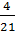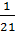### Sample ProblemUse your graphing calculator to construct a histogram showing the probability distribution for X, where X is a random variable that represents the sum when you took two balls from the balls above randomly. Based on this histogram, what is the probability of getting a 4, 7, or 11? Enter your number as an improper, irreducible, fraction in the form “a/b”.

/

#### Solution

The histogram shows that 4 has a probability of7 has a probability of, and 11 has a probability of. Thus, the probability of getting one of these sums is.